# Understanding Transformers, the Data Science Way

Read this accessible and conversational article about understanding transformers, the data science way — by asking a lot of questions that is.

Transformers have become the defacto standard for any NLP tasks nowadays. Not only that, but they are now also being used in Computer Vision and to generate music. I am sure you would all have heard about the GPT3 Transformer and its applications thereof. But all these things aside, they are still hard to understand as ever.

It has taken me multiple readings through the Google research paper that first introduced transformers along with just so many blog posts to really understand how a transformer works.

So, I thought of putting the whole idea down in as simple words as possible along with some very basic Math and some puns as I am a proponent of having some fun while learning. I will try to keep both the jargon and the technicality to a minimum, yet it is such a topic that I could only do so much. And my goal is to make the reader understand even the goriest details of Transformer by the end of this post.

Also, this is officially my longest post both in terms of time taken to write it as well as the length of the post. Hence, I will advise you to Grab A Coffee. ☕️

So, here goes — This post will be a highly conversational one and it is about “Decoding The Transformer”.

Q: So, Why should I even understand Transformer?

In the past, the LSTM and GRU architecture(as explained here in my past post on NLP) along with attention mechanism used to be the State of the Art Approach for Language modeling problems (put very simply, predict the next word) and Translation systems. But, the main problem with these architectures is that they are recurrent in nature, and the runtime increases as the sequence length increases. That is, these architectures take a sentence and process each word in a sequential way, and hence with the increase in sentence length the whole runtime increases.

Transformer, a model architecture first explained in the paper Attention is all you need, lets go of this recurrence and instead relies entirely on an attention mechanism to draw global dependencies between input and output. And that makes it FAST.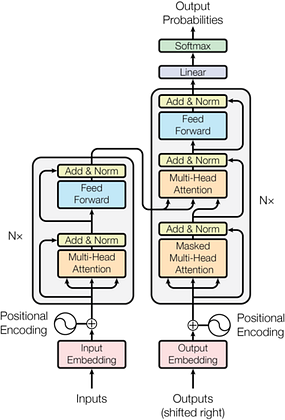This is the picture of the full transformer as taken from the paper. And, it surely is intimidating. So, I will aim to demystify it in this post by going through each individual piece. So read ahead.

### The Big Picture

Q: That sounds interesting. So, what does a transformer do exactly?

Essentially, a transformer can perform almost any NLP task. It can be used for language modeling, Translation, or Classification as required, and it does it fast by removing the sequential nature of the problem. So, the transformer in a machine translation application would convert one language to another, or for a classification problem will provide the class probability using an appropriate output layer.

It all will depend on the final outputs layer for the network but, the Transformer basic structure will remain quite the same for any task. For this particular post, I will be continuing with the machine translation example.

So from a very high place, this is how the transformer looks for a translation task. It takes as input an English sentence and returns a German sentence.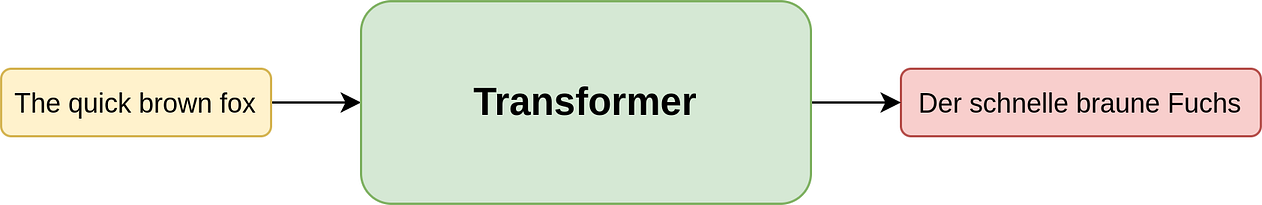Transformer for Translation (Image by author)

### The Building Blocks

Q: That was too basic. 😎 Can you expand on it?

Okay, just remember in the end, you asked for it. Let’s go a little deeper and try to understand what a transformer is composed of.

So, a transformer is essentially composed of a stack of encoder and decoder layers. The role of an encoder layer is to encode the English sentence into a numerical form using the attention mechanism, while the decoder aims to use the encoded information from the encoder layers to give the German translation for the particular English sentence.

In the figure below, the transformer is given as input an English sentence, which gets encoded using 6 encoder layers. The output from the final encoder layer then goes to each decoder layer to translate English to German.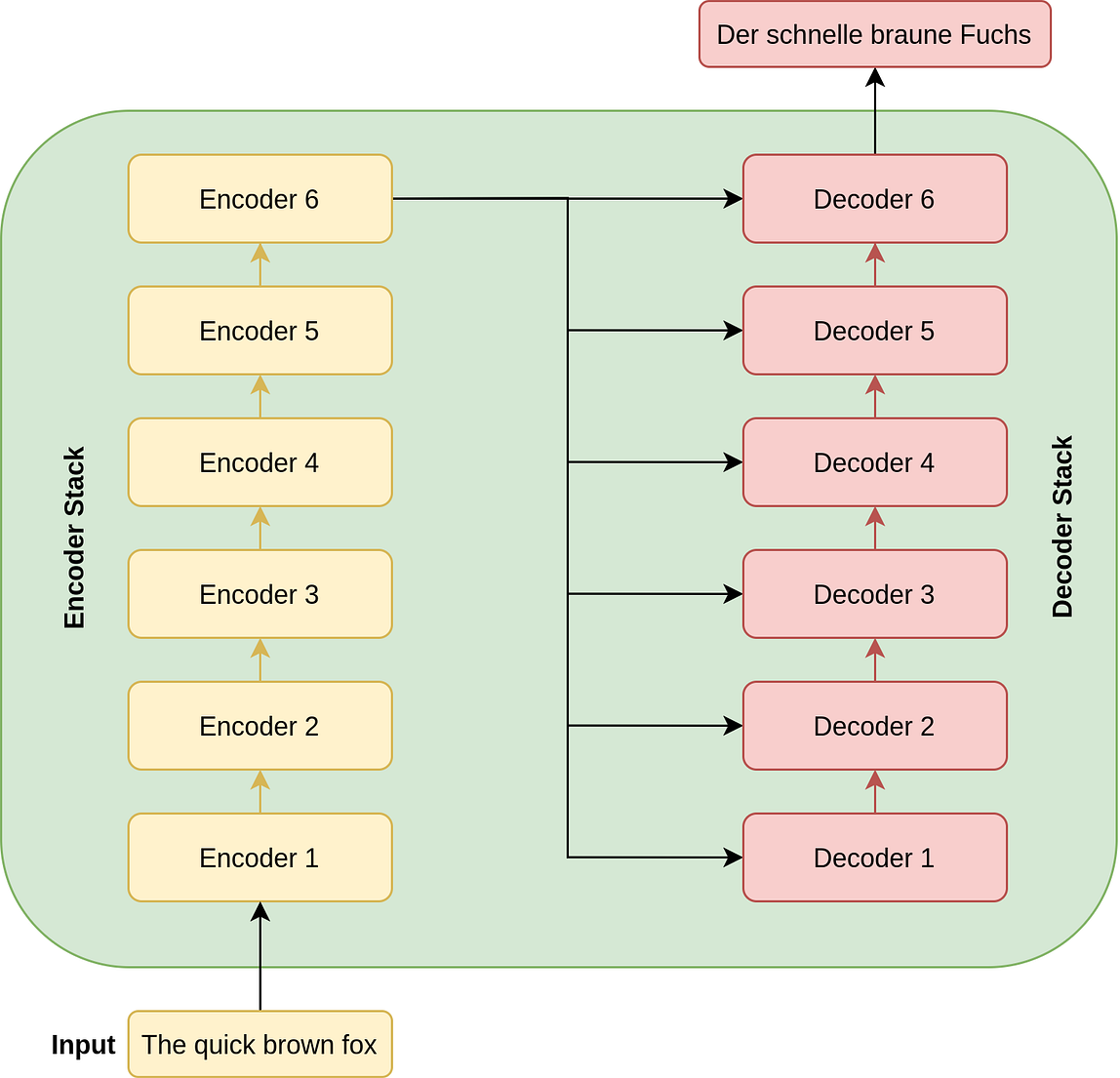Data Flow in a Transformer (Image by author)

### 1. Encoder Architecture

Q: That’s alright but, how does an encoder stack encode an English sentence exactly?

Patience, I am getting to it. So, as I said the encoder stack contains six encoder layers on top of each other(As given in the paper, but the future versions of transformers use even more layers). And each encoder in the stack has essentially two main layers:

• a multi-head self-attention Layer, and
• a position-wise fully connected feed-forward network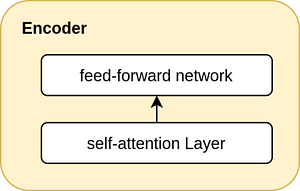Very basic encoder Layer (Image by author)

They are a mouthful. Right? Don’t lose me yet as I will explain both of them in the coming sections. Right now, just remember that the encoder layer incorporates attention and a position-wise feed-forward network.

Q: But, how does this layer expect its inputs to be?

This layer expects its inputs to be of the shape `SxD` (as shown in the figure below) where `S` is the source sentence(English Sentence) length, and `D` is the dimension of the embedding whose weights can be trained with the network. In this post, we will be using `D` as 512 by default throughout. While S will be the maximum length of sentence in a batch. So it normally changes with batches.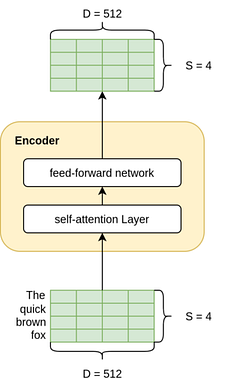Encoder — Input and Output shapes are the same (Image by author)

And what about the outputs of this layer? Remember that the encoder layers are stacked on top of each other. So, we want to be able to have an output of the same dimension as the input so that the output can flow easily into the next encoder. So the output is also of the shape, `SxD`.

Q: Enough about the sizes talk, I understand what goes in and what goes out but what actually happens in the Encoder layer?

Okay, let’s go through the attention layer and the feedforward layer one by one:

### A) Self-attention layer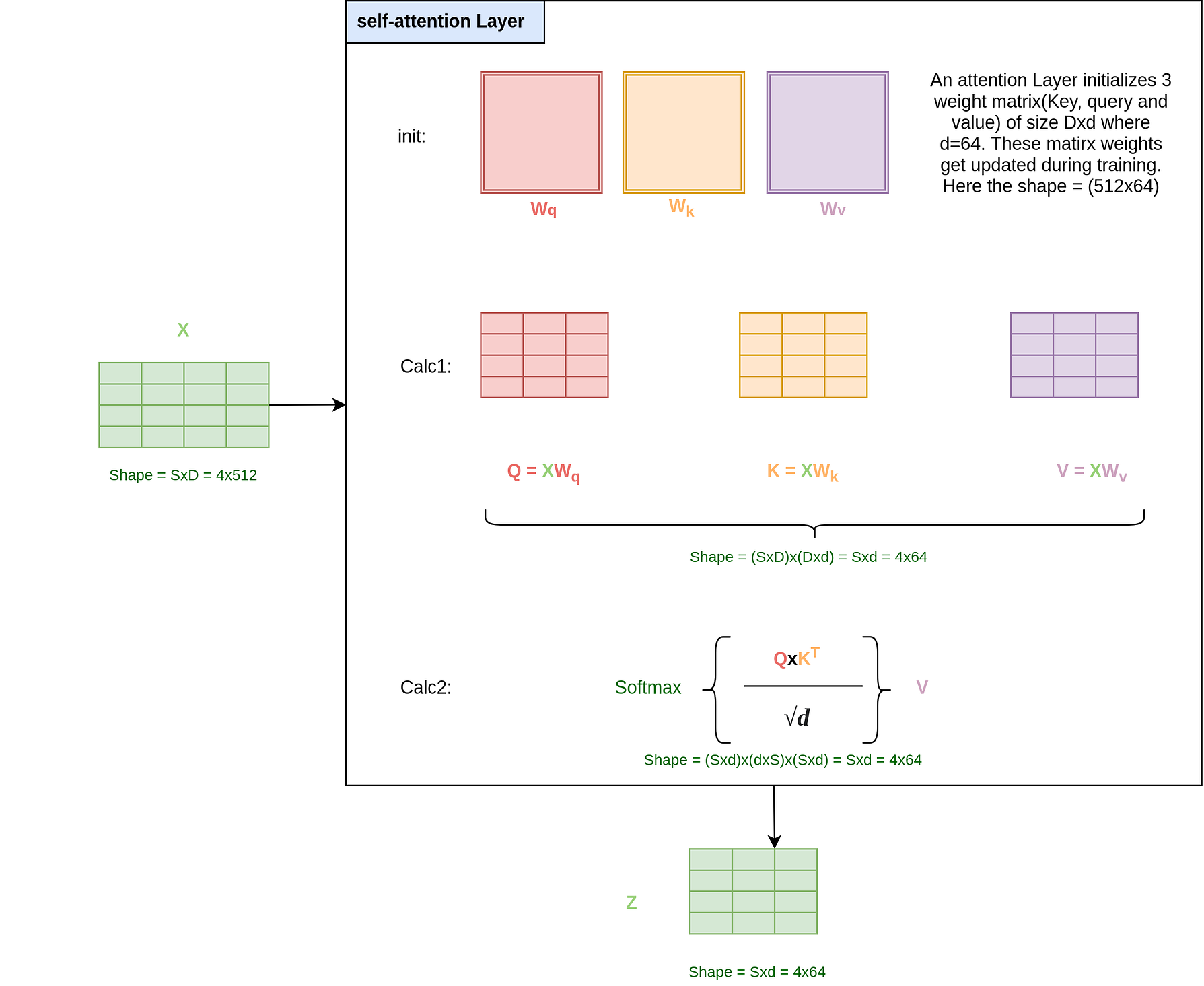How Self-Attention Works (Image by author)

The above figure must look daunting but it is easy to understand. So just stay with me here.

Deep Learning is essentially nothing but a lot of matrix calculations and what we are essentially doing in this layer is a lot of matrix calculations intelligently. The self-attention layer initializes with 3 weight matrices — Query(W_q), Key(W_k), and Value(W_v). Each of these matrices has a size of (`Dxd`) where d is taken as 64 in the paper. The weights for these matrices will be trained when we train the model.

In the first calculation(Calc 1 in the figure), we create matrices Q, K, and V by multiplying the input with the respective Query, Key, and Value matrix.

Till now it is trivial and shouldn’t make any sense, but it is at the second calculation where it gets interesting. Let’s try to understand the output of the softmax function. We start by multiplying the Q and Kᵀ matrix to get a matrix of size (`SxS`) and divide it by the scalar √d. We then take a softmax to make the rows sum to one.

Intuitively, we can think of the resultant `SxS` matrix as the contribution of each word in another word. For example, it might look like this: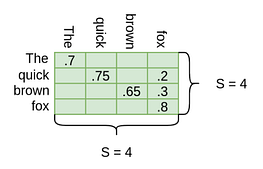Softmax(QxKt/sqrt(d)) (Image by author)

As you can see the diagonal entries are big. This is because the word contribution to itself is high. That is reasonable. But we can see here that the word “quick” devolves into “quick” and “fox” and the word “brown” also devolves into “brown” and “fox”. That intuitively helps us to say that both the words — “quick” and “brown” each refers to the “fox”.

Once we have this SxS matrix with contributions we multiply this matrix by the Value matrix(Sxd) of the sentence and it gives us back a matrix of shape Sxd(4x64). So, what the operation actually does is that it replaces the embedding vector of a word like “quick” with say .75 x (quick embedding) and .2x(fox embedding) and thus now the resultant output for the word “quick” has attention embedded in itself.

Note that the output of this layer has the dimension (Sxd) and before we get done with the whole encoder we need to change it back to D=512 as we need the output of this encoder as the input of another encoder.

Q: But, you called this layer Multi-head self-attention Layer. What is the multi-head?

Okay, my bad but in my defense, I was just getting to that.

It’s called a multi-head because we use many such self-attention layers in parallel. That is, we have many self-attention layers stacked on top of each other. The number of attention layers,h, is kept as 8 in the paper. So the input X goes through many self-attention layers parallelly, each of which gives a z matrix of shape (Sxd) = 4x64. We concatenate these 8(h) matrices and again apply a final output linear layer, Wo, of size DxD.

What size do we get? For the concatenate operation we get a size of SxD(4x(64x8) = 4x512). And multiplying this output by Wo, we get the final output Z with the shape of SxD(4x512) as desired.

Also, note the relation between h,d, and D i.e. h x d = D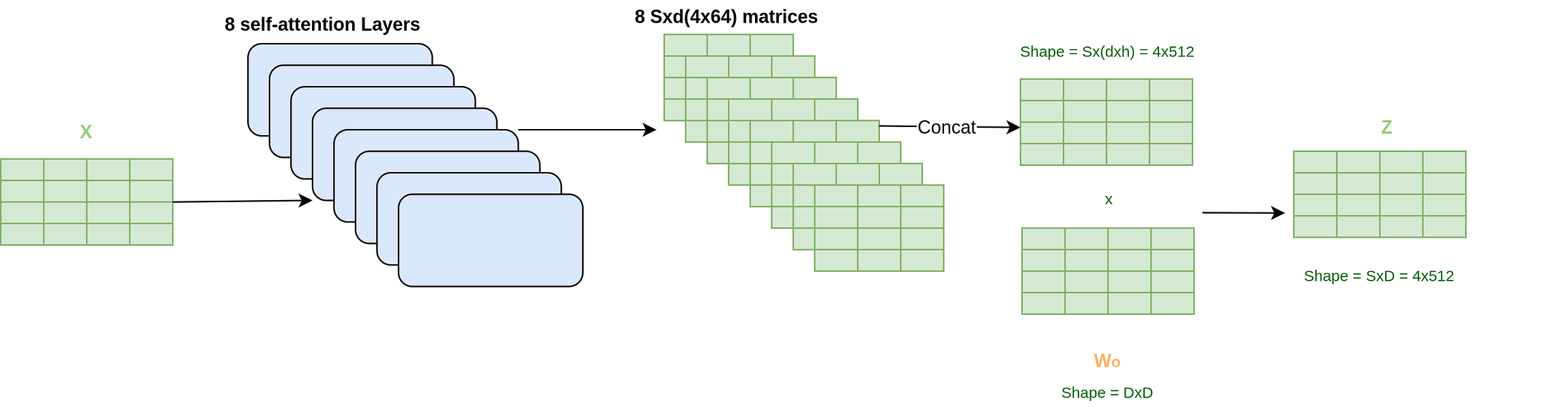The Full multi-headed self-attention Layer (Image by author)

Thus, we finally get the output Z of shape 4x512 as intended. But before it goes into another encoder we pass it through a Feed-Forward Network.

### B) Position-wise feed-forward network

Once we understand the multi-headed attention layer, the Feed-forward network is actually pretty easy to understand. It is just a combination of various linear and dropout layers on the output Z. Consequentially, it is again just a lot of Matrix multiplication here.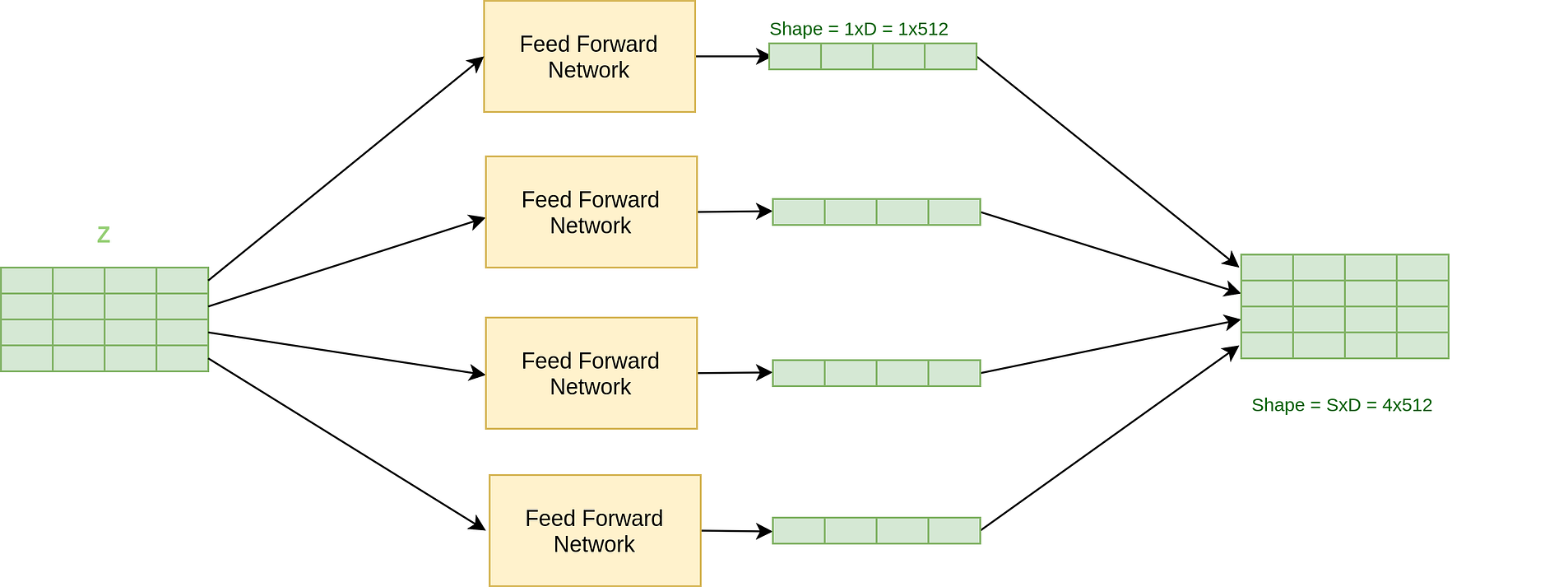Each word goes into the feed-forward network. (Image by author)

The feed-forward network applies itself to each position in the output Z parallelly(Each position can be thought of as a word) and hence the name Position-wise feed-forward network. The feed-forward network also shares weight, so that the length of the source sentence doesn’t matter(Also, if it didn’t share weights, we would have to initialize a lot of such networks based on max source sentence length and that is not feasible)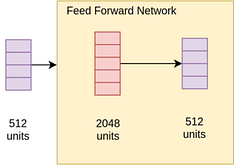It is actually just a linear layer that gets applied to each position(or word) (Image by author)

With this, we near an okayish understanding of the encoder part of the Transformer.

Q: Hey, I was just going through the picture in the paper, and the encoder stack has something called “positional encoding” and “Add & Norm” also. What are these?I am back again here so you don’t have to scroll Source

Okay, These two concepts are pretty essential to this particular architecture. And I am glad you asked this one. So, we will discuss these steps before moving further to the decoder stack.

### C. Positional Encodings

Since, our model contains no recurrence and no convolution, in order for the model to make use of the order of the sequence, we must inject some information about the relative or absolute position of the tokens in the sequence. To this end, we add “positional encodings” to the input embeddings at the bottoms of both the encoder and decoder stacks(as we will see later). The positional encodings need to have the same dimension, D as the embeddings have so that the two can be summed.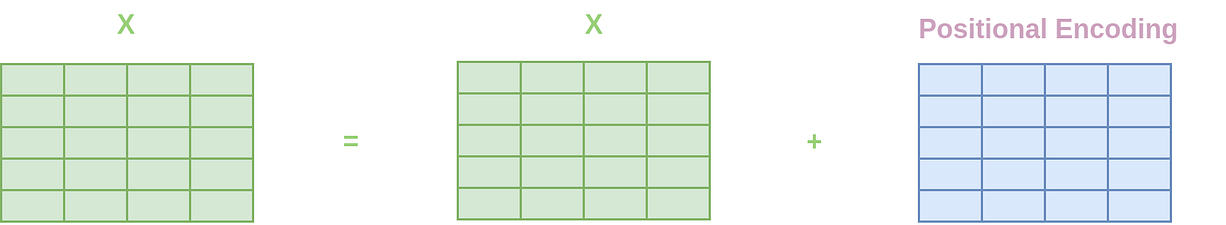Add a static positional pattern to X (Image by author)

In the paper, the authors used sine and cosine functions to create positional embeddings for different positions.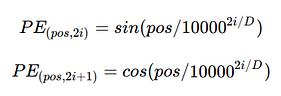This particular mathematical thing actually generates a 2d matrix which is added to the embedding vector that goes into the first encoder step.

Put simply, it’s just a constant matrix that we add to the sentence so that the network could get the position of the word.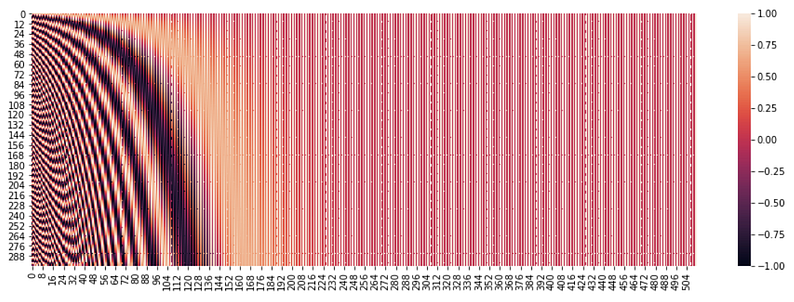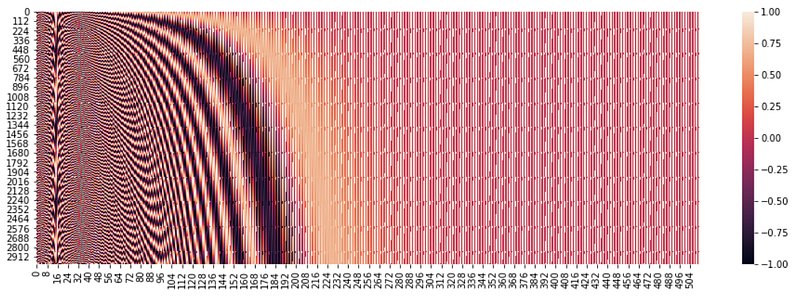Positional encoding matrix for the first 300 and 3000 positions (Image by author)

Above is the heatmap of the position encoding matrix that we will add to the input that is to be given to the first encoder. I am showing the heatmap for the first 300 positions and the first 3000 positions. We can see that there is a distinct pattern that we provide to our Transformer to understand the position of each word. And since we are using a function comprised of sin and cos, we are able to embed positional embeddings for very high positions also pretty well as we can see in the second picture.

Interesting Fact: The authors also let the Transformer learn these encodings too and didn’t see any difference in performance as such. So, they went with the above idea as it doesn’t depend on sentence length and so even if the test sentence is bigger than train samples, we would be fine.

Another thing, that I didn’t mention for the sake of simplicity while explaining the encoder is that the encoder(the decoder architecture too) architecture has skip level residual connections(something akin to resnet50) also. So, the exact encoder architecture in the paper looks like below. Simply put, it helps traverse information for a much greater length in a Deep Neural Network. This can be thought of as akin(intuitively) to information passing in an organization where you have access to your manager as well as to your manager’s manager.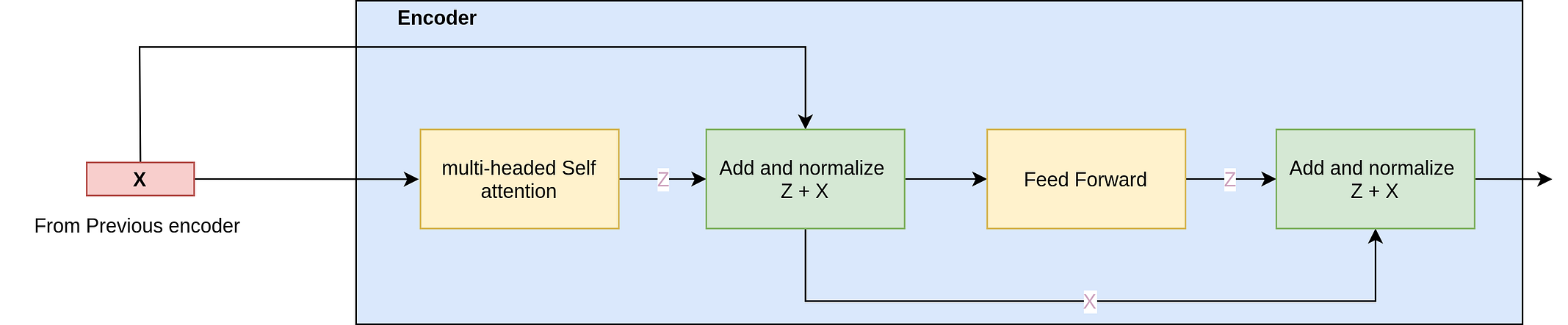The Skip level connections help information flow in the network (Image by author)

### 2. Decoder Architecture

Q: Okay, so till now we have learned that an encoder takes an input sentence and encodes its information in a matrix of size SxD(4x512). That’s all great but how does it help the decoder decode it to German?

Good things come to those who wait. So, before understanding how the decoder does that, let us understand the decoder stack.

The decoder stack contains 6 decoder layers in a stack (As given in the paper again) and each decoder in the stack is comprised of these main three layers:

• a position-wise fully connected feed-forward network

It also has the same positional encoding as well as the skip level connection as well. We already know how the multi-head attention and feed-forward network layers work, so we will get straight into what is different in the decoder as compared to the encoder.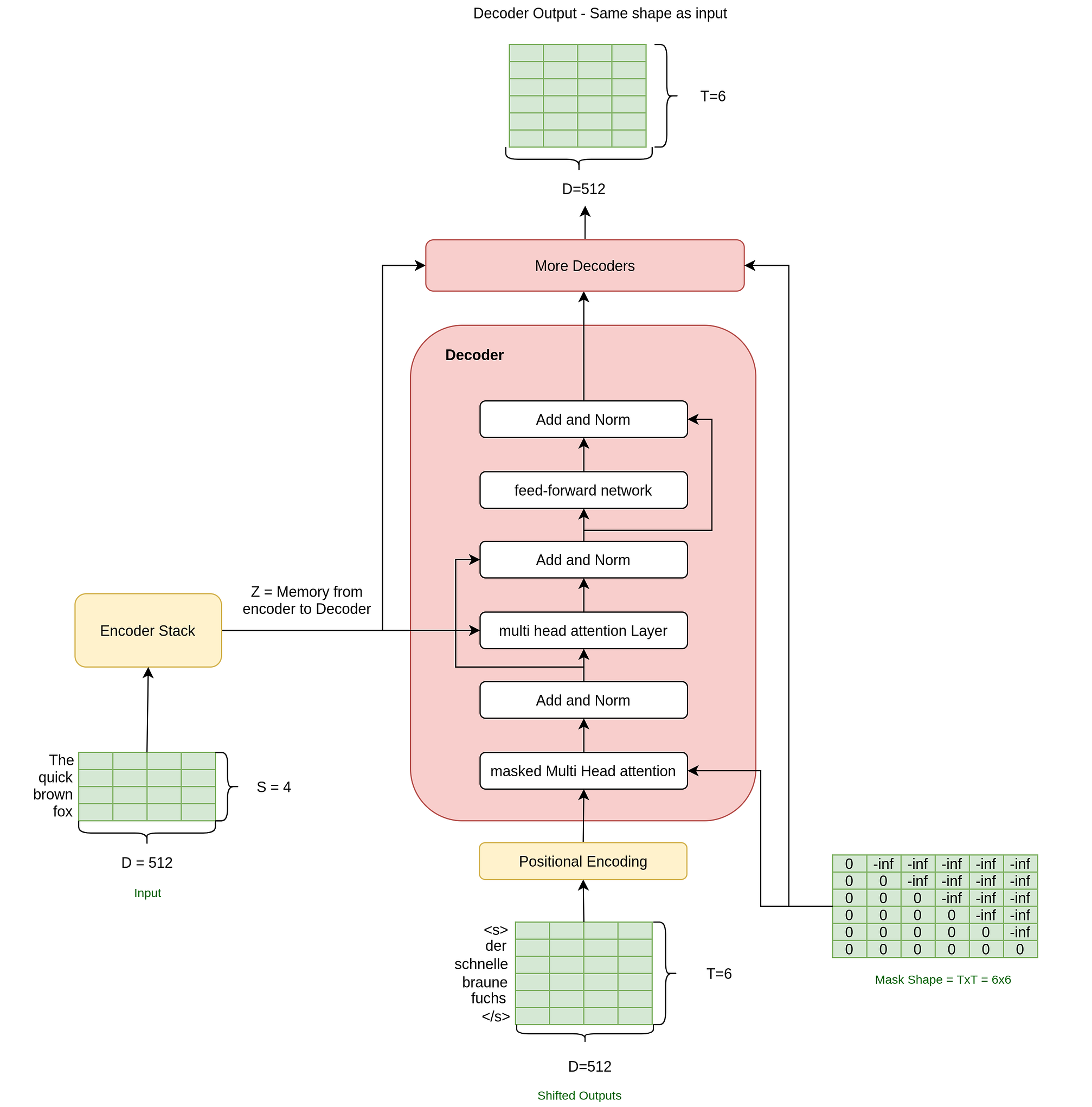Decoder Architecture (Image by author)

Q: Wait, but do I see the output we need flowing into the decoder as input? What? Why? 😖

I am noticing that you are getting pretty good at asking questions. And that is a great question, something I even though myself a lot of times, and something that I hope will get much clearer by the time you reach the end of this post.

But to give an intuition, we can think of a transformer as a conditional language model in this case. A model that predicts the next word given an input word and an English sentence on which to condition upon or base its prediction on.

Such models are inherently sequential as in how would you train such a model? You start by giving the start token(`<s>`) and the model predicts the first word conditioned on the English sentence. You change the weights based on if the prediction is right or wrong. Then you give the start token and the first word (`<s> der`) and the model predicts the second word. You change weights again. And so on.

The transformer decoder learns just like that but the beauty is that it doesn’t do that in a sequential manner. It uses masking to do this calculation and thus takes the whole output sentence (although shifted right by adding a `<s>` token to the front) while training. Also, please note that at prediction time we won’t give the output to the network

Q: But, how does this masking exactly work?

It works, as usual, you wear it I mean 😷. Kidding aside, as you can see that this time we have a Masked Multi-Head attention Layer in our decoder. This means that we will mask our shifted output (that is the input to the decoder) in a way that the network is never able to see the subsequent words since otherwise, it can easily copy that word while training.

So, how does the mask exactly work in the masked attention layer? If you remember, in the attention layer we multiplied the query(Q) and keys(K) and divided them by sqrt(d) before taking the softmax.

In a masked attention layer, though, we add the resultant matrix before the softmax(which will be of shape (TxT)) to a masking matrix.

So, In a masked layer, the function changes from: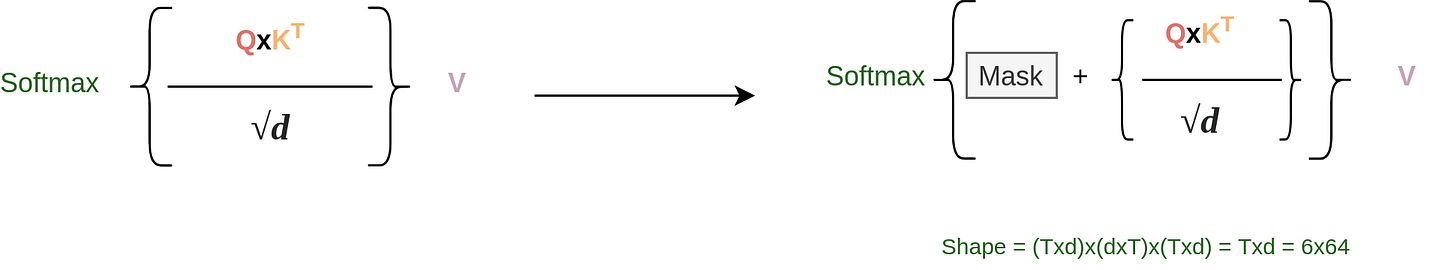(Image by author)

Q: I still don’t get it, what happens if we do that?

That’s understandable actually. Let me break it in steps. So, our resultant matrix(QxK/sqrt(d)) of shape (TxT) might look something like below:(The numbers can be big as softmax not applied yet)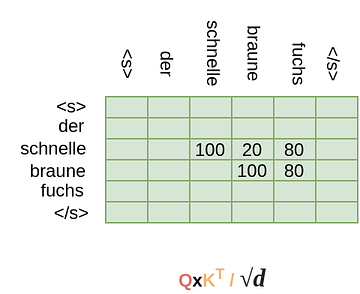Schnelle currently attends to both Braune and Fuchs (Image by author)

The word Schnelle will now be composed of both Braune and Fuchs if we take the above matrix’s softmax and multiply it with the value matrix V. But we don’t want that, so we add the mask matrix to it to give: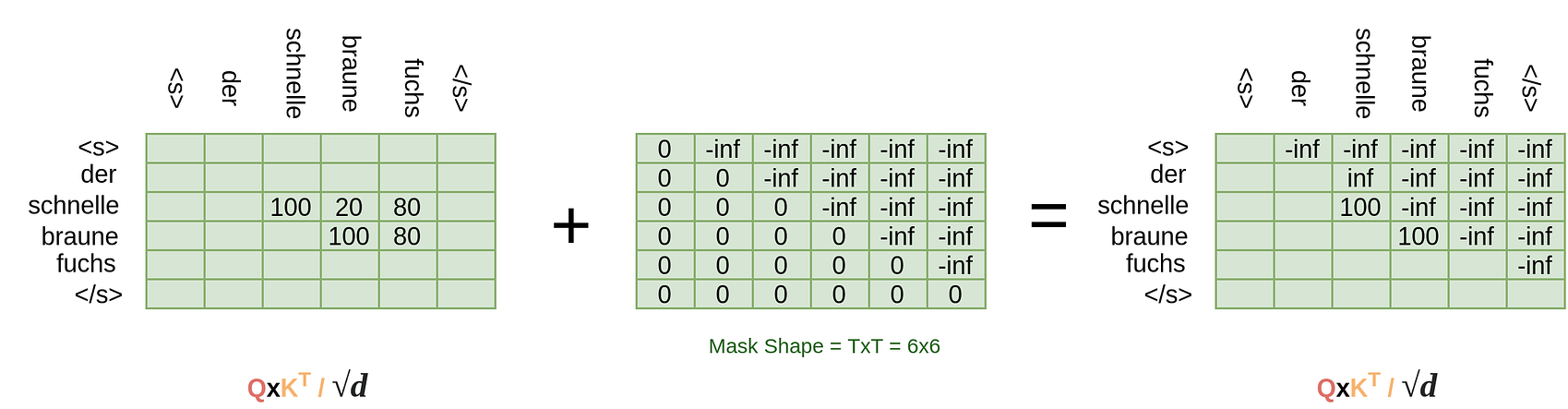The mask operation applied to the matrix. (Image by author)

And, now what will happen after we do the softmax step?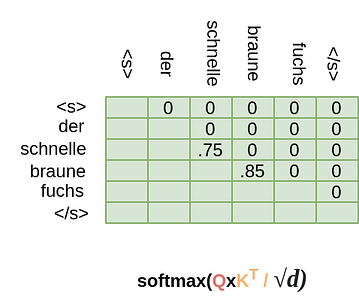Schnelle never attends to any word after Schnelle. (Image by author)

Since e^{-inf} = 0, all positions subsequent to Schnelle have been converted to 0. Now, if we multiply this matrix with the value matrix V, the vector corresponding to Schnelle’s position in the Z vector passing through the decoder would not contain any information of the subsequent words Braune and Fuchs just like we wanted.

And that is how the transformer takes the whole shifted output sentence at once and doesn’t learn in a sequential manner. Pretty neat I must say.

Q: Are you kidding me? That’s actually awesome.

So glad that you are still with me and you appreciate it. Now, coming back to the decoder. The next layer in the decoder is:

As you can see in the decoder architecture, a Z vector(Output of encoder) flows from the encoder to the multi-head attention layer in the Decoder. This Z output from the last encoder has a special name and is often called as memory. The attention layer takes as input both the encoder output and data flowing from below(shifted outputs) and uses attention. The Query vector Q is created from the data flowing in the decoder, while the Key(K) and value(V) vectors come from the encoder output.

Q: Isn’t there any mask here?

No, there is no mask here. The output coming from below is already masked and this allows every position in the decoder to attend over all the positions in the Value vector. So for every word position to be generated the decoder has access to the whole English sentence.

Here is a single attention layer(which will be part of a multi-head just like before):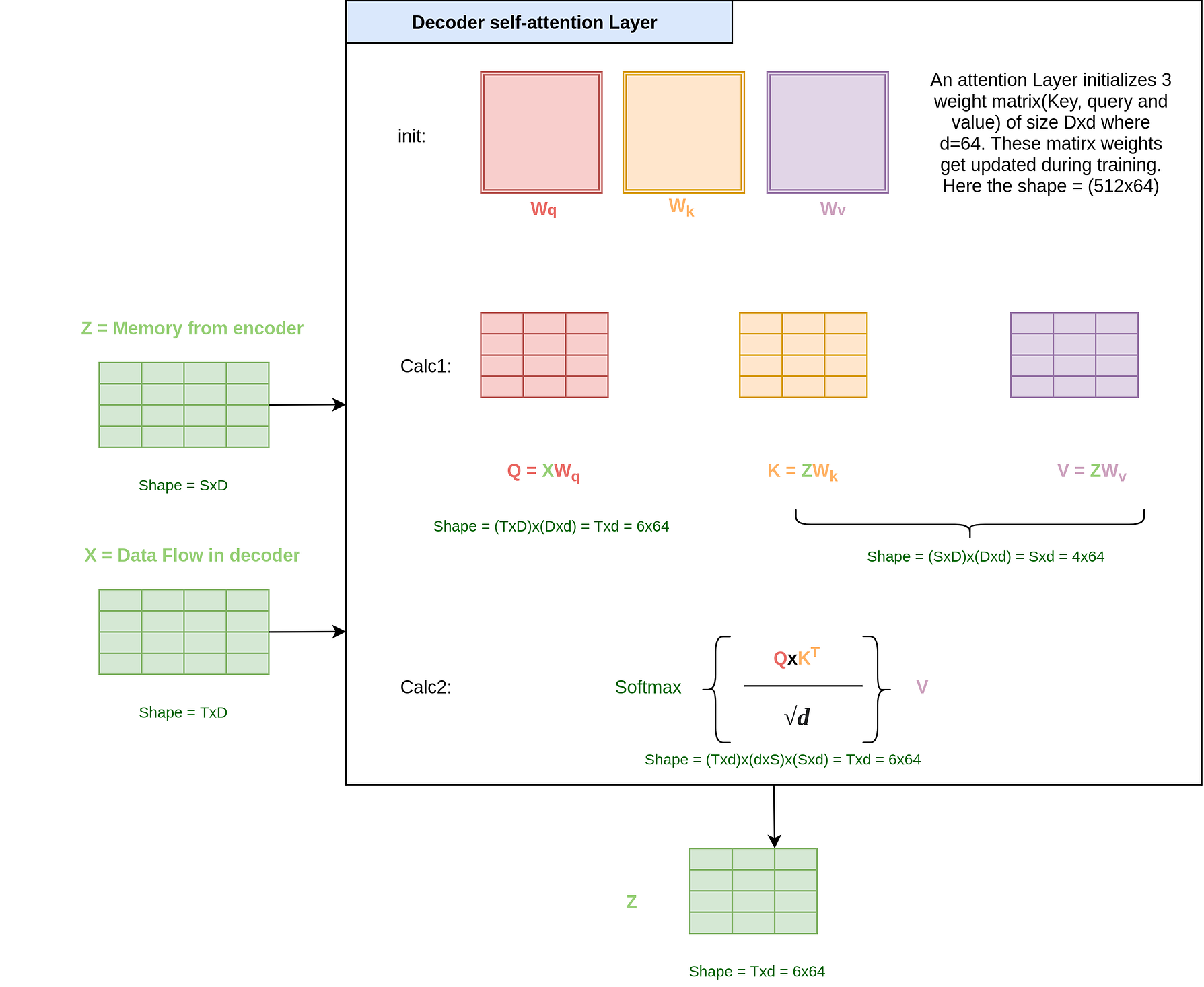(Image by author)

Q: But won’t the shapes of Q, K, and V be different this time?

You can look at the figure where I have done all the weights calculation. I would also ask you to see the shapes of the resultant Z vector and how our weight matrices until now never used the target or source sentence length in any of their dimensions. Normally, the shape cancels away in all our matrix calculations. For example, see how the S dimension cancels away in calculation 2 above. That is why while selecting the batches during training the authors talk about tight batches. That is in a batch all source sentences have similar lengths. And different batches could have different source lengths.

I will now talk about the skip level connections and the feed-forward layer. They are actually the same as in ….

Q: Ok, I get it. We have the skip level connections and the FF layer and get a matrix of shape TxD after this whole decode operation. But where is the German translation?

We are actually very much there now friend. Once, we are done with the transformer, the next thing is to add a task-specific output head on the top of the decoder output. This can be done by adding some linear layers and softmax on top to get the probability across all the words in the german vocab. We can do something like this: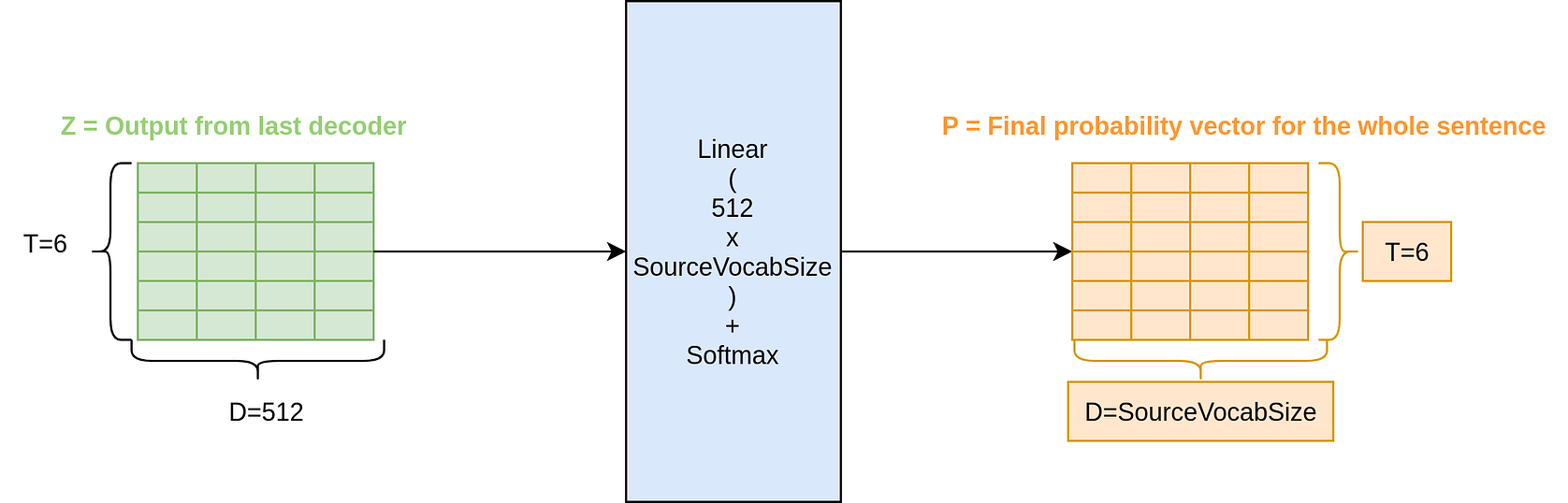(Image by author)

As you can see we are able to generate probabilities. So far we know how to do a forward pass through this Transformer architecture. Let us see how we do the training of such a Neural Net Architecture.

### Training:

Till now, if we take a bird-eye view of the structure we have something like: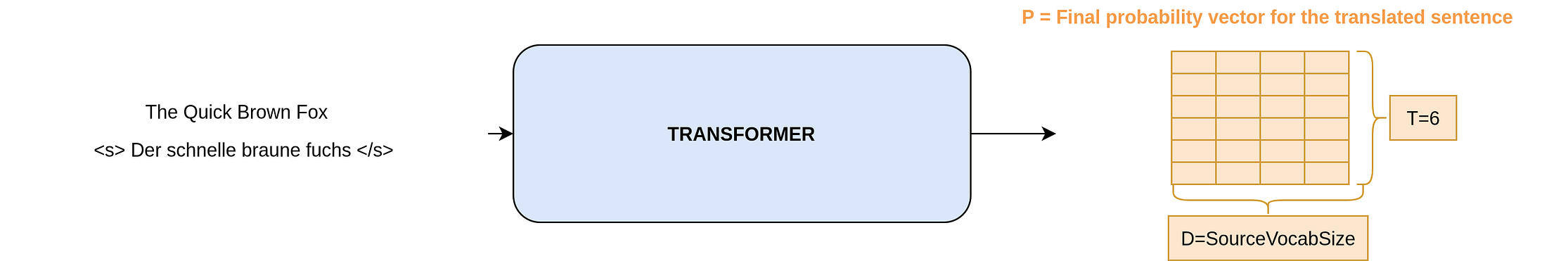(Image by author)

We can give an English sentence and shifted output sentence and do a forward pass and get the probabilities over the German vocabulary. And thus we should be able to use a loss function like cross-entropy where the target could be the German word we want, and train the neural network using the Adam Optimizer. Just like any classification example. So, there is your German.

In the paper though, the authors use slight variations of optimizers and loss. You can choose to skip the below 2 sections on KL Divergence Loss and Learning rate schedule with Adam if you want as it is done only to churn out more performance out of the model and not an inherent part of the Transformer architecture as such.

Q: I have been here for such a long time and have I complained? 😒

Okay. Okay. I get you. Let’s do it then.

### A) KL Divergence with Label Smoothing:

KL Divergence is the information loss that happens when the distribution P is approximated by the distribution Q. When we use the KL Divergence loss, we try to estimate the target distribution(P) using the probabilities(Q) we generate from the model. And we try to minimize this information loss in the training.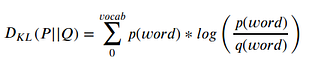(Image by author)

If you notice, in this form(without label smoothing which we will discuss) this is exactly the same as cross-entropy. Given two distributions like below.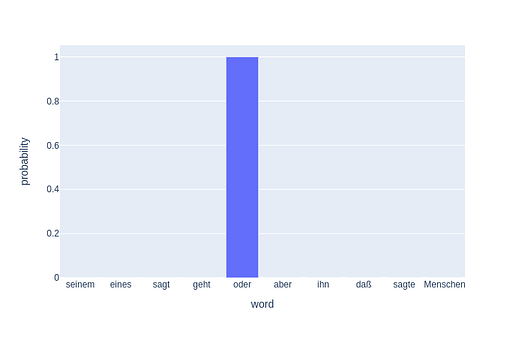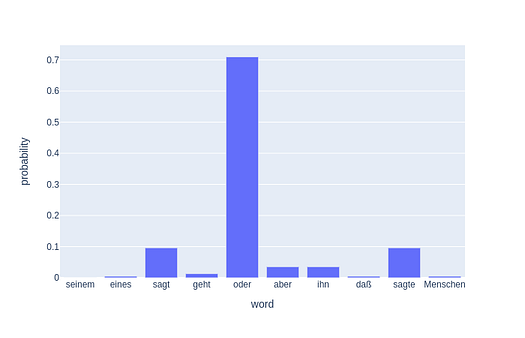Target distribution and probability distribution for a word(token) (Image by author)

The KL Divergence formula just plain gives `-logq(oder)` and that is the cross-entropy loss.

In the paper, though the authors used label smoothing with α = 0.1 and so the KL Divergence loss is not cross-entropy. What that means is that in the target distribution the output value is substituted by (1-α) and the remaining 0.1 is distributed across all the words. The authors say that this is so that the model is not too confident.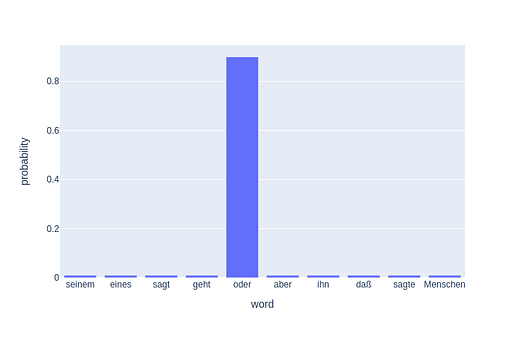(Image by author)

Q: But, why do we make our models not confident? It seems absurd.

Yes, it does but intuitively, you can think of it as when we give the target as 1 to our loss function, we have no doubts that the true label is True and others are not. But vocabulary is inherently a non-standardized target. For example, who is to say that you cannot use good in place of great? So we add some confusion in our labels so our model is not too rigid.

### B) A particular Learning Rate schedule with Adam

The authors use a learning rate scheduler to increase the learning rate until warmup steps and then decrease it using the below function. And they used the Adam optimizer with β¹ = 0.9, β² = 0.98. Nothing too interesting here just some learning choices.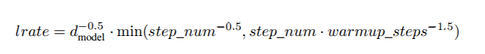Source: Paper

Q: But wait I just remembered that we won’t have the shifted output at the prediction time, would we? How do we do predictions then?

If you realize what we have at this point is a generative model and we will have to do the predictions in a generative way as we won’t know the output target vector when doing prediction. So predictions are still sequential.

### Prediction TimePredicting with a greedy search using the Transformer (Image by author)

This model does piece-wise predictions. In the original paper, they use the Beam Search to do prediction. But a greedy search would work fine as well for the purpose of explaining it. In the above example, I have shown how a greedy search would work exactly. The greedy search would start with:

• Passing the whole English sentence as encoder input and just the start token `<st> `as shifted output(input to the decoder) to the model and doing the forward pass.
• The model will predict the next word — `der`
• Then, we pass the whole English sentence as encoder input and add the last predicted word to the shifted output(input to the decoder = `<st> der`) and do the forward pass.
• The model will predict the next word — `schnelle`
• Passing the whole English sentence as encoder input and `<st> der schnelle` as shifted output(input to the decoder) to the model and doing the forward pass.
• and so on, until the model predicts the end token `</s>` or we generate some maximum number of tokens(something we can define) so the translation doesn’t run for an infinite duration in any case it breaks.

### Beam Search:

Q: Now I am greedy, Tell me about beam search as well.

Okay, the beam search idea is inherently very similar to the above idea. In beam search, we don’t just look at the highest probability word generated but the top two words.

So, For example, when we gave the whole English sentence as encoder input and just the start token as shifted output, we get two best words as `i`(p=0.6) and `der`(p=0.3). We will now generate the output model for both output sequences,`<s> i` and `<s> der` and look at the probability of the next top word generated. For example, if `<s> i` gave a probability of (p=0.05) for the next word and `<s> der>` gave (p=0.5) for the next predicted word, we discard the sequence `<s> i`and go with `<s> der` instead, as the sum of probability of sentence is maximized(`<s> der next_word_to_der` p = 0.3+0.5 compared to `<s> i next_word_to_i` p = 0.6+0.05). We then repeat this process to get the sentence with the highest probability.

Since we used the top 2 words, the beam size is 2 for this Beam Search. In the paper, they used beam search of size 4.

PS: I showed that the English sentence is passed at every step for brevity, but in practice, the output of the encoder is saved and only the shifted output passes through the decoder at each time step.

Q: Anything else you forgot to tell me? I will let you have your moment.

Yes. Since you asked. Here it is:

### BPE, Weight Sharing and Checkpointing

In the paper, the authors used Byte pair encoding to create a common English German vocabulary. They then used shared weights across both the English and german embedding and pre-softmax linear transformation as the embedding weight matrix shape would work (Vocab Length X D).

Also, the authors average the last k checkpoints to create an ensembling effect to reach the performance. This is a pretty known technique where we average the weights in the last few epochs of the model to create a new model which is sort of an ensemble.

Q: Can you show me some code?

This post has already been so long, so I will do that in the next post. Stay tuned.

Now, finally, my turn to ask the question: Did you get how a transformer works? Yes, or No, you can answer in the comments. :)

References

In this post, I covered how the Transformer architecture works from a detail-oriented, intuitive perspective.

If you want to learn more about NLP, I would like to call out an excellent course on Natural Language Processing from the Advanced Machine Learning Specialization. Do check it out.

I am going to be writing more of such posts in the future too. Let me know what you think about them. Should I write on heavily technical topics or more beginner level articles? The comment section is your friend. Use it. Also, follow me up at Medium or Subscribe to my blog.

And, finally a small disclaimer — There might be some affiliate links in this post to relevant resources, as sharing knowledge is never a bad idea.

This story was first published here.

Bio: Rahul Agarwal is Senior Statistical Analyst at WalmartLabs. Follow him on Twitter @mlwhiz.

Original. Reposted with permission.

Related: